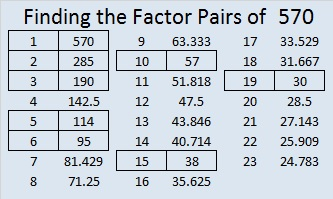# 570 and Level 6

570 is the hypotenuse of Pythagorean triple 342-456-570. What 3 digit number is the greatest common factor of those three numbers?Print the puzzles or type the solution on this excel file: 12 Factors 2015-07-27

—————————————————————————————————

• 570 is a composite number.
• Prime factorization: 570 = 2 x 3 x 5 x 19
• The exponents in the prime factorization are 1, 1, 1, and 1. Adding one to each and multiplying we get (1 + 1)(1 + 1)(1 + 1)(1 + 1) = 2 x 2 x 2 x 2 = 16. Therefore 570 has exactly 16 factors.
• Factors of 570: 1, 2, 3, 5, 6, 10, 15, 19, 30, 38, 57, 95, 114, 190, 285, 570
• Factor pairs: 570 = 1 x 570, 2 x 285, 3 x 190, 5 x 114, 6 x 95, 10 x 57, 15 x 38, or 19 x 30
• 570 has no square factors that allow its square root to be simplified. √570 ≈ 23.87467—————————————————————————————————